# Animatable LED Counters, Clocks, Etc, Using The Material Room

#### 3dcheapskate

While trying to get a grip on the Du, Dv, dPdu, dPdv, dNdu and dNdv I created a very simplistic 'ten digit visualizer' in the Poser material room. The MT5 is posted on ShareCG here Ten Digit Visualizer (Poser MT5) - Poser - ShareCG if anybody wants to play.

In the comments on that ShareCG page English Bob pointed me at a thread where he, bagginsbill and some others had already created something similar in this "Animation in material files?" thread at Renderosity - www.renderosity.com/mod/forumpro/?thread_id=2852563

In the first page of that thread bagginsbill demonstrates how to display a single digit from an image containing all the digits 0 to 9, and shows several different display styles.

On the second page English Bob show show to use a number from another node (e.g. Frame_Number) as the input, and display a single digit from that number.

And they both obviously worked out how to display multiple digits - the end results were shown, but not the shader trees.

Since I was beginning to go crazy with Du, Dv, dPdu, dPdv, dNdu and dNdv I decided to try and work that out for myself...

(screenshot should appear in the next post)

#### 3dcheapskate

Here's what I came up with. It's not a complete shader, more a 'shader snippet' showing the principle.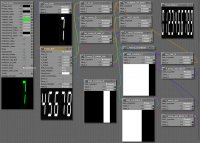Most of the nodes are simple 'Math_Functions', but I used the 'Rename Node'wacro (can't remember where that came from) to give them more meaningful names.

There are three inputs that control the display:

N__NUMBER_OF_DIGITS - This is the number of digits you want across the width of the texture. I've set 5 for a 5-digit display.
P__DIGIT_POSITION - This decides which of the N digits the shader snippet is displaying. 1 is the first (leftmost), 2 is the second from left, etc. I'veset this to 4 because I want the fourth digit, i.e. second from right.
D__DIGIT_VALUE - This is the digit value I want to display at position P of the N-digit display. I've set this to 7 - I want the fourth digit of my 5 digit display to be 7.

Note: 'Image_Map_2' in the top right corner is not connected, and is just there for comparison. The 'Image_Map' node uses the same image, but has mathematical equations driving the U_Scale and U_Offset node. I then apply a mask to this scaled and offset image to extract the digit I want at the correct position.

•Pendraia

#### English Bob

And they both obviously worked out how to display multiple digits - the end results were shown, but not the shader trees.

If you want multiple digits, you simply clone as many copies of the digit as you need. Then change Value_2 in Math_Functions_3 for each digit according to its significance - 1 for the units (least significant) digit, 10 for the tens and so on. The example screenshot shows the hundreds digit.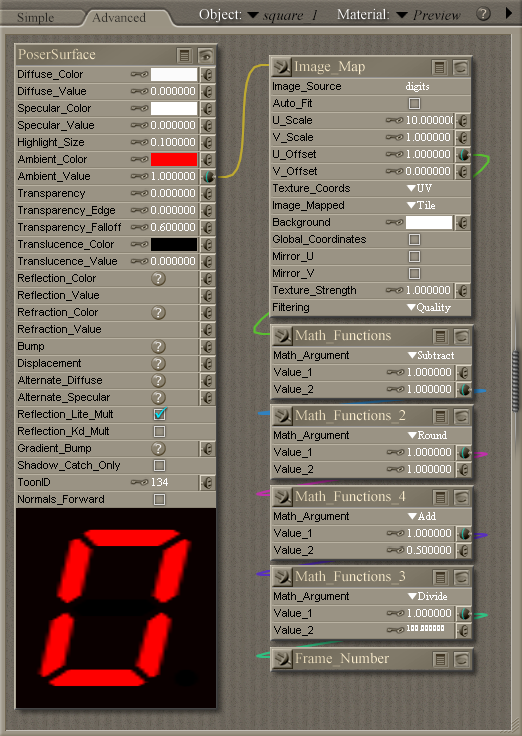#### 3dcheapskate

:facepalm: Of course ! You're using a separate prop for each digit ! Looking at your thread again you started with a single digit LED prop, so you used the same shader for each, but with different weighting values (units, tens, etc)

I came at this from a different direction - I wanted all the digits in a single material. Using a 10 x 1 strip (each digit 0 to 9 appearing once) seemed a better approach than my 10x10 grid with each digit duplicated ten times. If I wanted to be able to display up to ten digits with the partial shader I posted here I would need to duplicate (for each additional digit) every node except PoserSurface, N__NUMBER_OF_DIGITS, and the unconnected Image_Map_2. I'd then have to extract each D__DIGIT_VALUE from Frame_Number (or whatever node I'm trying to display the value of) using a network similar to yours. And I'd also have to determine each P__DIGIT_POSITION...

I see a ballpark figure of around 200 nodes ?

I'm beginning to like the single-digit material ! And as a general purpose thing I can see it being far more useful. But for my very specific test purpose, I really want ten digits in a single material.

#### English Bob

Sure, understood. The single digit is simple, but it does require the value you want displayed to be globally accessible to every one of the digits somehow - easy for an already global quantity such as frame number, not so much otherwise.

You can theoretically expand this approach, both by increasing the number of positions on the strip in powers of ten, and by making it two-dimensional with additional nodes to drive V_Offset. But I doubt you'd stretch it to ten digits.

#### 3dcheapskate

I'm actually making some progress using your number strip and expanding the network I posted above. I've tagged a (blank) and a '-' onto the end of your digit strip and got those displaying as the first 'digit' of a positive/negative number respectively.

I'm now working on extracting the individual digit positions and values from a numeric output (e.g. Frame_Number, Math_Functions) to use in place of each P__DIGIT_POSITION and D__DIGIT_VALUE. I found a beautifully simple pseudo-mathematical way to get the number of significant digits before the decimal point for any number x:

log10(abs(x))+1 rounded down to the nearest integer

...except that I've run into rounding/accuracy problems with values of x that are very fractionally below integer powers of ten. I've posted a query about that over in the CGbytes Node Knows forum.

#### English Bob

I replied to your post at CGBytes, but for reference the node values are represented in IEEE 754 floating point.

This convertor will explain for those who are curious and don't want to forum-hop: IEEE 754 Converter

#### 3dcheapskate

Thanks - that link explains it very nicely.

Assuming that it was going to be something inherent in the binary representation of the numbers, I realized that I could probably work around this with a few extra nodes. I think I have that bit worked out now.

(P.S. the '...I found a beautifully simple pseudo-mathematical way...' in my previous post should really have been '...I found a beautifully simple mathematical way...' - the 'pseudo-mathematical' part simply being the way my calculation was written)

#### English Bob

Since it's Star Wars Day I wondered about saying, in my best Yoda accent, "Do mathematics, or do no mathematics. There is no pseudo-mathematics."#### 3dcheapskate

May the 4th be with you ! You are truly wise Master Bob !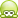#### 3dcheapskate

Here's the additional batch of nodes for calculating C (the number of integer digits required to represent the number x), plus a workaround for catching cases when rounding causes an error (numbers very slightly below integer powers of ten - e.g. x=99.999985 gives C=3 instead of 2)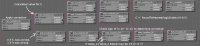The basic calculation for the number of integer digits is this:
C = RoundToNearest(Log10(Abs(X))+0.5)

To double-check that I've calculated C correctly I use the fact that 10^(C-1) is the smallest number that requires C integer digits. So if C has been correctly calculated (x-10^(C-1)) should be positive - or zero if x is an exact integer power of ten, e.g. 1, 10, 100,etc. If C has been incorrectly calculated it will be exactly 1 too big, so I simply subtract 1. If C was correct I subtract nothing.

The new problem is that zero can be +ve or -ve in IEEE754. So if x is an exact integer power of ten my workaround may get things wrong. So I now need a workaround for the workaround...

Isn't this fun !Notes:
In post #6 my pseudo-mathematically-written calculation stated "rounded down to the nearest integer". But the Round function of the Math_Functions node is actually a round-to-nearest, so I have to add 0.5 instead of 1 before doing the round.
Also the Log function of the Math_Functions node is actually the natural log (log to base e) not the log to base 10. So I used log10(x) = ln(x)/ln(10) to get round that.
And yes, ln(10) has accuracy issues

#### 3dcheapskate

Using a copy of the (slightly modified) shader snippet from post 2 for each of 9 digits, another copy of it with a few more changes to put a +/- sign at the start, and with the calculation of the number of integer digits required from post 11, I now have a shader that will display signed integers from -999,999,999 to +999,999,999 adjusting the width of each digit so that the signed number matches the full width of the UV map.

204 nodes. I was going to upload it but I've just realized that I need to fix the paths, set image filtering to 'None', etc, collapse a few nodes, etc. So not just yet.

The following weren't intentional, but just happened with the way I stuck things together:
- Anything after the decimal point is ignored.
- A zero input results in nothing on the output - it's just black.
- A positive value less than 1 results in just the plus sign being displayed, nothing else.
- A negative value with magnitude less than one results in just the minus sign being displayed
- If the number requires more than 9 digits the digit widths will be adjusted as if the 10th, 11th, etc digits were there - but they'll just be blank.

And of course those IEEE754 rounding errors and signed zeroes are still a problem...

Anyway, here's a render of nine squares with exactly the same 204 node shader applied. I've just changed one value in each: -1234567890, -123456, -123, -0.123, 0, 0.123, 123, 123456, and 1234567890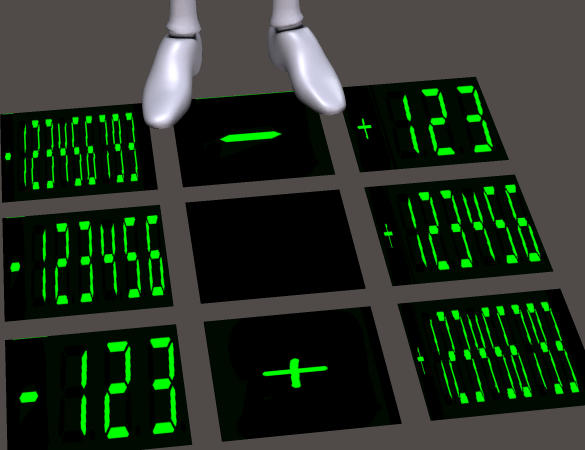Next step, digits beyond the decimal point perhaps?

(Thinking several steps ahead, how about a vector visualizer - three numbers in red, green and blue?)